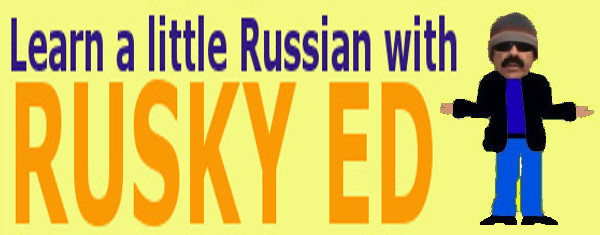Arithmetic - Miscellaneous
[ Number Sense ]    [ Addition and Subtraction ]    [ Multiplication and Division ]    [ Factors and Multiples ]    [ Decimal Operations ]    [ Fraction Operations ]    [ Miscellaneous ]    [ Home ]
 FEATURED VIDEOS:
```
```

## Arithmetic - Miscellaneous - Perimeter of Triangles

Perimeter is the distance around a shape. Consider this triangle:As you can see it has three sides which are 13, 10, and 16 units long. To find the total distance around this object you simply add up the length of each side.

```
PERIMETER = 13 + 10 + 16 = 39 units

```
Here's another triangle for you to inspect.As you can see it has three sides which are 7, 11, and 10 units long. To find the total distance around this object you simply add up the length of each side.
```
PERIMETER = 11 + 10 + 7 = 28 units

```
Remember units can be inches, feet, yards, meters, or any other unit of length. We use the term units when the actual unit is left unspecified.
Custom Search
[ Number Sense ]    [ Addition and Subtraction ]    [ Multiplication and Division ]    [ Factors and Multiples ]    [ Decimal Operations ]    [ Fraction Operations ]    [ Miscellaneous ]    [ Home ]